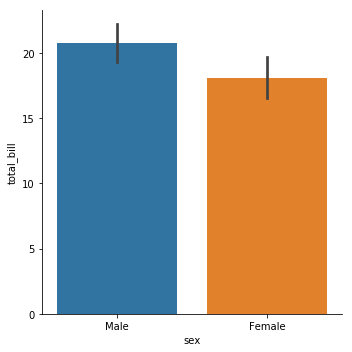In :
#Import library
import seaborn as sns
%matplotlib inline

In :
tips = sns.load_dataset('tips')

Out:
total_bill tip sex smoker day time size
0 16.99 1.01 Female No Sun Dinner 2
1 10.34 1.66 Male No Sun Dinner 3
2 21.01 3.50 Male No Sun Dinner 3
3 23.68 3.31 Male No Sun Dinner 2
4 24.59 3.61 Female No Sun Dinner 4

## barplot and countplot¶

These very similar plots allow you to get aggregate data off a categorical feature in your data. barplot is a general plot that allows you to aggregate the categorical data based off some function, by default the mean:

In :
sns.barplot(x='sex',y='total_bill',data=tips)

C:\ProgramData\Anaconda3\lib\site-packages\scipy\stats\stats.py:1713: FutureWarning: Using a non-tuple sequence for multidimensional indexing is deprecated; use arr[tuple(seq)] instead of arr[seq]. In the future this will be interpreted as an array index, arr[np.array(seq)], which will result either in an error or a different result.
return np.add.reduce(sorted[indexer] * weights, axis=axis) / sumval

Out:
<matplotlib.axes._subplots.AxesSubplot at 0x9900eb8>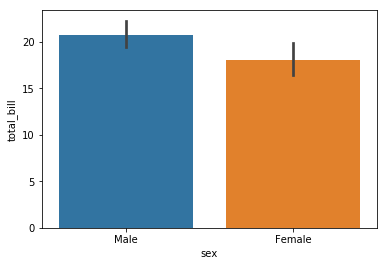In :
import numpy as np


You can change the estimator object to your own function, that converts a vector to a scalar:

In :
sns.barplot(x='sex',y='total_bill',data=tips,estimator=np.std)

Out:
<matplotlib.axes._subplots.AxesSubplot at 0x9a0fc50>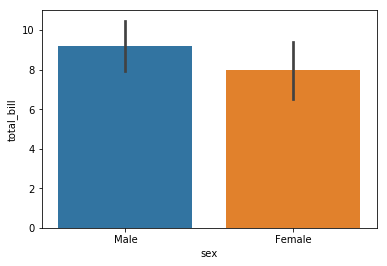### countplot¶

This is essentially the same as barplot except the estimator is explicitly counting the number of occurrences. Which is why we only pass the x value:

In :
sns.countplot(x='sex',data=tips)

Out:
<matplotlib.axes._subplots.AxesSubplot at 0x9a6cb00>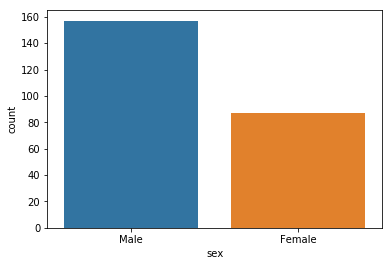## boxplot and violinplot¶

boxplots and violinplots are used to shown the distribution of categorical data. A box plot (or box-and-whisker plot) shows the distribution of quantitative data in a way that facilitates comparisons between variables or across levels of a categorical variable. The box shows the quartiles of the dataset while the whiskers extend to show the rest of the distribution, except for points that are determined to be “outliers” using a method that is a function of the inter-quartile range.

In :
sns.boxplot(x="day", y="total_bill", data=tips,palette='rainbow')

Out:
<matplotlib.axes._subplots.AxesSubplot at 0xaaa2668>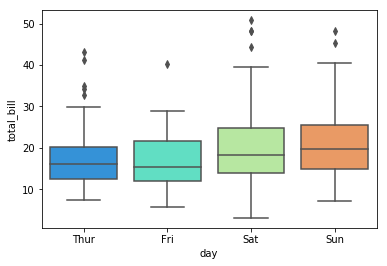In :
# Can do entire dataframe with orient='h'
sns.boxplot(data=tips,palette='rainbow',orient='h')

Out:
<matplotlib.axes._subplots.AxesSubplot at 0xab2f898>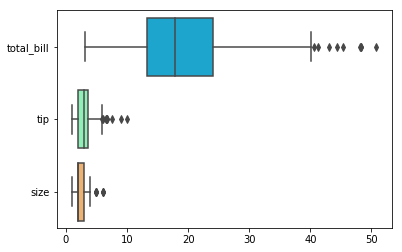In :
sns.boxplot(x="day", y="total_bill", hue="smoker",data=tips, palette="coolwarm")

Out:
<matplotlib.axes._subplots.AxesSubplot at 0xaba1ef0>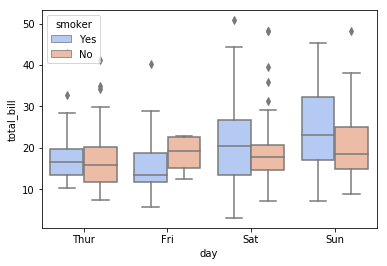### violinplot¶

A violin plot plays a similar role as a box and whisker plot. It shows the distribution of quantitative data across several levels of one (or more) categorical variables such that those distributions can be compared. Unlike a box plot, in which all of the plot components correspond to actual datapoints, the violin plot features a kernel density estimation of the underlying distribution.

In :
sns.violinplot(x="day", y="total_bill", data=tips,palette='rainbow')

Out:
<matplotlib.axes._subplots.AxesSubplot at 0xb094e48>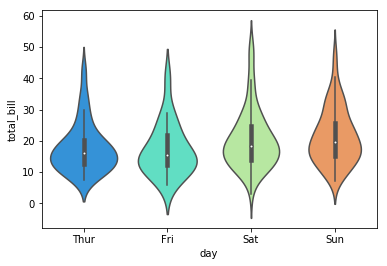In :
sns.violinplot(x="day", y="total_bill", data=tips,hue='sex',palette='Set1')

Out:
<matplotlib.axes._subplots.AxesSubplot at 0xb0ed0b8>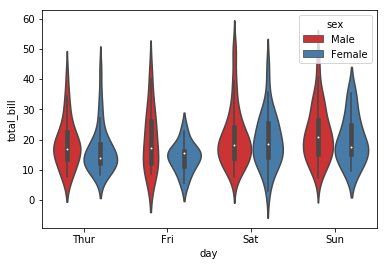In :
sns.violinplot(x="day", y="total_bill", data=tips,hue='sex',split=True,palette='Set1')

Out:
<matplotlib.axes._subplots.AxesSubplot at 0xac36a20>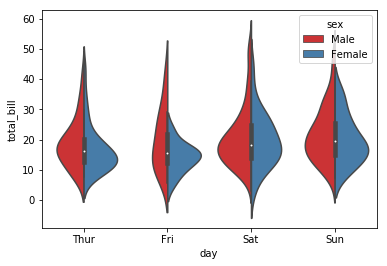## stripplot and swarmplot¶

The stripplot will draw a scatterplot where one variable is categorical. A strip plot can be drawn on its own, but it is also a good complement to a box or violin plot in cases where you want to show all observations along with some representation of the underlying distribution.

The swarmplot is similar to stripplot(), but the points are adjusted (only along the categorical axis) so that they don’t overlap. This gives a better representation of the distribution of values, although it does not scale as well to large numbers of observations (both in terms of the ability to show all the points and in terms of the computation needed to arrange them).

In :
sns.stripplot(x="day", y="total_bill", data=tips)

Out:
<matplotlib.axes._subplots.AxesSubplot at 0xacc45c0>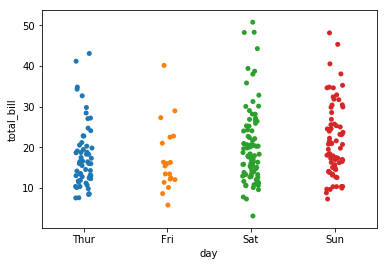In :
sns.stripplot(x="day", y="total_bill", data=tips,jitter=True)

Out:
<matplotlib.axes._subplots.AxesSubplot at 0xad15f60>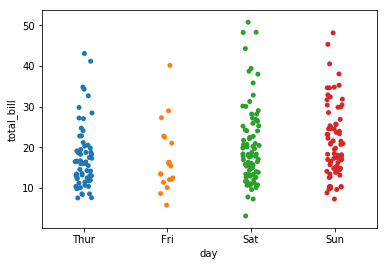In :
sns.stripplot(x="day", y="total_bill", data=tips,jitter=True,hue='sex',palette='Set1')

Out:
<matplotlib.axes._subplots.AxesSubplot at 0xad6bb70>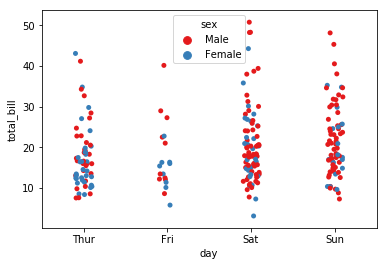In :
sns.stripplot(x="day", y="total_bill", data=tips,jitter=True,hue='sex',palette='Set1',split=True)

C:\ProgramData\Anaconda3\lib\site-packages\seaborn\categorical.py:2775: UserWarning: The split parameter has been renamed to dodge.
warnings.warn(msg, UserWarning)

Out:
<matplotlib.axes._subplots.AxesSubplot at 0xae7b588>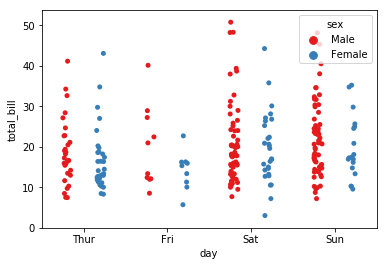In :
sns.swarmplot(x="day", y="total_bill", data=tips)

Out:
<matplotlib.axes._subplots.AxesSubplot at 0xaf1fb38>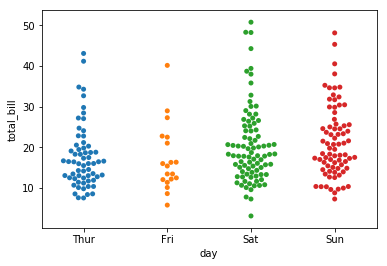In :
sns.swarmplot(x="day", y="total_bill",hue='sex',data=tips, palette="Set1", split=True)

C:\ProgramData\Anaconda3\lib\site-packages\seaborn\categorical.py:2974: UserWarning: The split parameter has been renamed to dodge.
warnings.warn(msg, UserWarning)

Out:
<matplotlib.axes._subplots.AxesSubplot at 0xaf7cb38>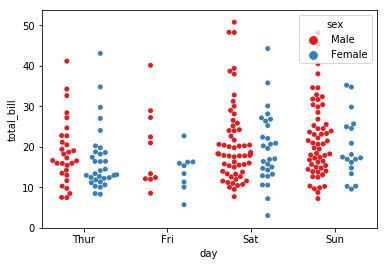### Combining Categorical Plots¶

In :
sns.violinplot(x="tip", y="day", data=tips,palette='rainbow')
sns.swarmplot(x="tip", y="day", data=tips,color='black',size=3)

Out:
<matplotlib.axes._subplots.AxesSubplot at 0xafdd048>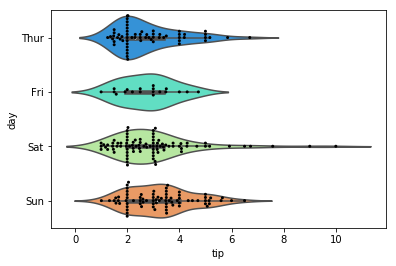## factorplot¶

factorplot is the most general form of a categorical plot. It can take in a kind parameter to adjust the plot type:

In :
sns.factorplot(x='sex',y='total_bill',data=tips,kind='bar')

C:\ProgramData\Anaconda3\lib\site-packages\seaborn\categorical.py:3666: UserWarning: The factorplot function has been renamed to catplot. The original name will be removed in a future release. Please update your code. Note that the default kind in factorplot ('point') has changed 'strip' in catplot.
warnings.warn(msg)

Out:
<seaborn.axisgrid.FacetGrid at 0x99ef358>